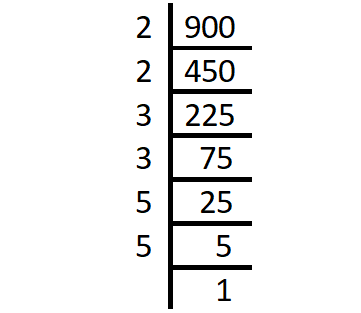Request a Tool

Prime Factor Calculator

Prime Factor Calculator is use to calculate prime factors of input number.

Prime Factor
0

Step1

Divide the given number by the smallest prime number. In this case, the smallest prime number should divide the number exactly

Step2

Again, divide the quotient by the smallest prime number

Step3

Repeat the process, until the quotient becomes 1.

ExampleDefination / Uses

Prime factors are factors of a number that are prime numbers in and of themselves. There are several methods for determining a number's prime factors, but one of the most prevalent is to use a prime factor tree.

A prime number is a whole number larger than 1 that can't be made by multiplying other whole numbers. Because of the fundamental theorem of arithmetic, prime numbers are commonly utilised in number theory. Natural numbers greater than 1 are either prime or may be factored as a product of prime numbers, according to the theorem. You can use the product of prime factors calculator to find the product of prime numbers since prime factorization is the decomposition of a composite number into a product of prime numbers.